Courses

# JEE(MAIN) Mathematics Mock Test

## 30 Questions MCQ Test JEE Main Mock Test Series 2020 & Previous Year Papers | JEE(MAIN) Mathematics Mock Test

Description
This mock test of JEE(MAIN) Mathematics Mock Test for JEE helps you for every JEE entrance exam. This contains 30 Multiple Choice Questions for JEE JEE(MAIN) Mathematics Mock Test (mcq) to study with solutions a complete question bank. The solved questions answers in this JEE(MAIN) Mathematics Mock Test quiz give you a good mix of easy questions and tough questions. JEE students definitely take this JEE(MAIN) Mathematics Mock Test exercise for a better result in the exam. You can find other JEE(MAIN) Mathematics Mock Test extra questions, long questions & short questions for JEE on EduRev as well by searching above.
QUESTION: 1

Solution:
QUESTION: 2

Solution:
QUESTION: 3

### The limiting point of the system of co-axial circles x2+y2-6x-6y+4=0, x2+y2-2x-4y+3=0 is

Solution:
QUESTION: 4

The are (in square units) of the region bounded by x2 = 8 y , x = 4 and x-axis is

Solution:
QUESTION: 5

The number of tangents drawn from the point (-1,2) to x2y2+2x-4y+4=0 is

Solution:

The correct option is Option D.

It is found that the centre of the circle is (-1,2)

Hence, no tangents would pass through it.

QUESTION: 6

The conjugate of complex number [(2+5i)/(4-3i)] is

Solution:
QUESTION: 7

[(1-i)/(1+i)] =

Solution:
QUESTION: 8

The solution of differential equation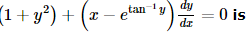Solution:
QUESTION: 9

The maximum value of the function f(x)=[(x)/(4+x+x2)] at the interval [-1,1] will be

Solution:
QUESTION: 10

sin⁻1 (cos(sin⁻1 x) + cos⁻1 (sin(cos⁻1 x) is equal to

Solution:
QUESTION: 11

In the following question, a Statement of Assertion (A) is given followed by a corresponding Reason (R) just below it. Read the Statements carefully and mark the correct answer-
Assertion(A): y = sin (ax + b) is a general solution of y" + a 2 y = 0 .
Reason(R) : y = sin (ax + b)  is a trigonometric function.

Solution:
QUESTION: 12

In the following question, a Statement of Assertion (A) is given followed by a corresponding Reason (R) just below it. Read the Statements carefully and mark the correct answer-
Assertion(A): The domain of the function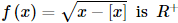Reason (R): The domain of the function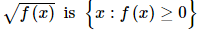Solution:
QUESTION: 13

The inverse of the converse of a conditional statement is the _______________.

Solution:

The inverse will insert NOT into the statement.
The converse will switch the IF and THEN.
The contrapositive does both.
The contrapositive is the inverse of the converse of the conditional statement.

QUESTION: 14

If A is a square matrix such that A2 = I, then A⁻1 is equal to

Solution:
QUESTION: 15

Two finite sets have m and n elements, the total number of subsets of the first set is 56 more than the total number of subsets of the second. The value of m and n are respectively

Solution:

Let A denote the first set and B denote the second set
We have, n(A) = 2m and n(B) = 2n
As per the question, we have
n(A) = 56 + n(B)
⇒ n(A) - n(B) = 56
⇒ 2m - 2n = 56
⇒ 2n (2m - n - 1)
⇒ 2n (2m - n - 1) = 8 x 7
⇒ 2n = 8 = 23 or (2m - n - 1) = 7
⇒ n = 3 or 2m - n = 8 = 23 = 26 - 3
⇒ n = 3 or m - n = 3
⇒ n = 3 or m = 6
Hence, the required values of m and n are 6 and 3 respectively

QUESTION: 16

The equation of the directrix to the parabola y2 − 2x − 6y − 5 = 0 is

Solution:
QUESTION: 17

A die is thrown 100 times. If getting an odd number is considered a success, the variance of the number of successes is

Solution:
QUESTION: 18

If in a moderately asymmetrical distribution mode and mean of the data are 6λ and 9λ respectively, then median is

Solution:
QUESTION: 19

The sides of a parallelogram are lx + ny + n = 0, lx + my + n' = 0, mx + ly + n = 0, mx + ly + n' = 0, the angle between its diagonals is

Solution:
QUESTION: 20
Equation of normal to the curve y = x(2 - x) at the point (2, 0) is
Solution:
QUESTION: 21
The direction ratios of the normal to the plane passing through the points (1, -2, 3), (-1, 2, -1) and parallel to (x - 2)/2 = (y + 1)/3 = z/4 is
Solution:
QUESTION: 22

If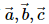are unit vector such that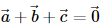then the value of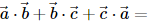=

Solution:
QUESTION: 23
If A+B+C=π, then cosB+cosC is equal to
Solution:
QUESTION: 24
Vector B=3j+4k, is equal to the sum of B₁ and B₂. If B₁ and B₂ are parallel and perpendicular to A=i+j, then B₁=
Solution:
QUESTION: 25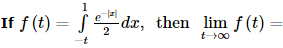Solution:
QUESTION: 26

The value of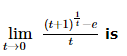Solution: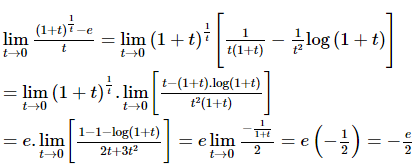QUESTION: 27

In the following question, a Statement of Assertion (A) is given followed by a corresponding Reason (R) just below it. Read the Statements carefully and mark the correct answer-
Assertion(A): A variable circle passes through the fixed point A(p,q) and touches x-axis . The locus of the other end of the diameter through A is (x - p)2 = 4qy.
Reason(R): An equation of the tangent to the circle x2 + y2 + 2gx + 2fy + c = 0 at the point (x1, y1) on the circle is xx1 - yy1 + g(x - x1) + f(y - y1) - c = 0 .

Solution:
QUESTION: 28

If A and B are the matrices of same order such that AB = A and BA = B, then A and B are

Solution:
QUESTION: 29

The largest value of 2x3- 3x2 - 12x + 5 for - 2 ≤ x ≤ 4 occurs at x =

Solution:
QUESTION: 30

Consider an infinite geometric series with first term a and common ratio r. If its sum is 4 and the second term is 3/4, then

Solution: